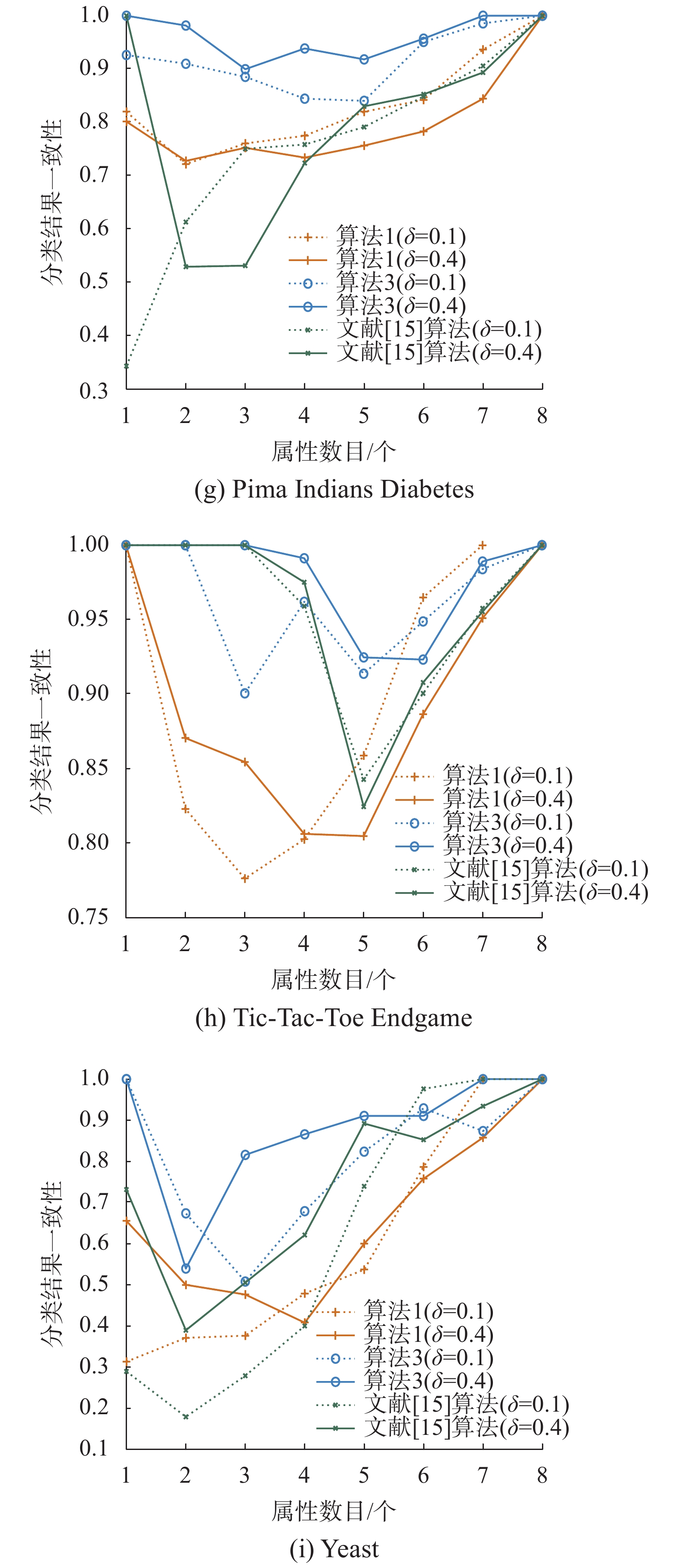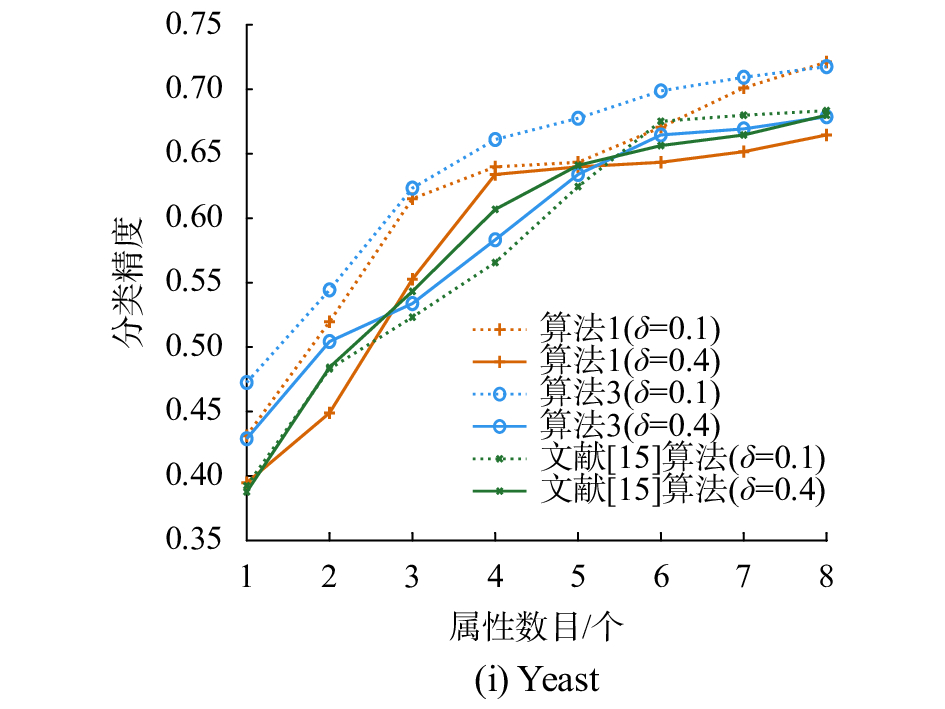﻿ 重要度集成的属性约简方法研究
«上一篇文章快速检索 高级检索

 智能系统学报2018, Vol. 13Issue (3): 414-421  DOI: 10.11992/tis.2017060800

引用本文LI Jingzheng, YANG Xibei, DOU Huili, et al. Research on ensemble significance based attribute reduction approach[J]. CAAI Transactions on Intelligent Systems, 2018, 13(3), 414-421. DOI: 10.11992/tis.201706080.文章历史

1. 江苏科技大学 计算机学院, 江苏 镇江 212003;
2. 南京理工大学 经济管理学院, 江苏 南京 210094;
3. 江苏科技大学 数理学院, 江苏 镇江 212003

Research on ensemble significance based attribute reduction approach
LI Jingzheng1, YANG Xibei1,2, DOU Huili1, WANG Pingxin3, CHEN Xiangjian1
1. School of Computer, Jiangsu University of Science and Technology, Zhenjiang 212003, China;
2. School of Economics and Management, Nanjing University of Science and Technology, Nanjing 210094, China;
3. School of Mathematics and Physics, Jiangsu University of Science and Technology, Zhenjiang 212003, China
Abstract: In the process of computing reduct using a heuristic algorithm, the attribute with the highest importance is gradually added in. However, this approach neglects the fluctuation of important calculations which is directly caused by data perturbation. Notably, such fluctuation may lead to an unstable reduct result. To eliminate such an anomaly, a framework consisting of a heuristic algorithm based on the importance of the ensemble attribute was proposed. In this approach, firstly, multiple sampling is executed for raw data; secondly, in each cycle, the importance of each attribute is computed on the basis of each sampling and the importance indices are integrated; finally, the attribute with the highest importance is added into the reduct. The experimental results obtained by utilizing the neighborhood rough set method show that the new approach not only obtains a more stable reduct, but also attains the classification results with high uniformity.
Key words: attribute reduction    classification    clustering    data perturbation    ensemble    heuristic algorithm    neighborhood rough set    stability

1 邻域粗糙集

 ${\rm{Int}}({x_i}) = \mathop {\min }\limits_{1 \leqslant j \leqslant n,j \ne i} {r_{ij}} + \delta \times (\mathop {\max }\limits_{1 \leqslant j \leqslant n,j \ne i} {r_{ij}} - \mathop {\min }\limits_{1 \leqslant j \leqslant n,j \ne i} {r_{ij}})$ (1)

 $\delta ({x_i}) = \{ {x_i} \in U|{x_j} \ne {x_i},{r_{ij}} \leqslant {\rm{Int}}({x_i})\}$ (2)

 $\underline {{N_B}} D = \bigcup\limits_{i{\rm{ = }}1}^N {\underline {{N_B}} {X_i}}$ (3)
 $\overline {{N_B}} D = \bigcup\limits_{i{\rm{ = }}1}^N {\overline {{N_B}} {X_i}}$ (4)

 $\underline {{N_B}} {X_i} = \{ {x_i} \in U|{\delta _B}({x_i}) \subseteq {X_i}\}$ (5)
 $\overline {{N_B}} {X_i} = \{ {x_i} \in U|{\delta _B}({x_i}) \cap {X_i} \ne \text{Ø} \}$ (6)

 $\gamma (B,D) = \frac{{{\rm{|}}\underline {{N_B}} D{\rm{|}}}}{{|U|}}$ (7)

2 属性约简 2.1 属性重要度与启发式算法

 ${\rm{Sig}}(a,B,D) = \gamma (B \cup \{ a\} ,D) - \gamma (B,D)$ (8)

1) seq←Ø，γ(seq, D)=0；

2) 若AT–seq = Ø，则转至5)，否则转至3)；

3) ${\rm {\forall}}$ ai∈AT–seq;

4) 选择aj，满足Sig(aj, seq, D)=max{Sig(ai, seq, D): ${\rm {\forall}}$ ai∈AT–seq}，令seq=seq∪{aj}，返回2),计算Sig(ai, seq, D)；

5) 输出seq。

2.2 集成属性重要度

1) ${U'} = \text{Ø}$

2) 利用k-means聚类获得U上的类簇C = $\{ {C_1},{C_2}, \cdots ,{C_N}\}$ ，其中N为决策类的个数；

3) for j = 1 to N

①计算类簇Cj中每个样本到类簇中心的平均距离 ${\overline d _j}$

②将Cj中到类簇中心的距离大于平均距离 ${\overline d _j}$ 的样本挑选出来加入 ${U'}$

end for

4) 输出 ${\rm{D}}{{\rm{S}}'}$

1) seq←Ø，γ(seq, D)=0；

2) for r = 1 to k

end for

3) 若AT–seq = Ø，则转至7)，否则转至4)；

4) for r = 1 to k

${\rm {\forall}}$ ai∈AT–seq，计算属性ai在决策系统DSr上的重要度Sigr(ai, AT, D)；

end for

5) ${\rm {\forall}}$ ai∈AT– seq，融合属性ai在各个决策系统上的重要度：

 ${\rm{Sig}}({a_i},{\rm{AT}},D) = \frac{{\displaystyle\sum\limits_{r = 1}^K {{\rm{Si}}{{\rm{g}}_r}({a_i},{\rm{AT}},D)} }}{k};$

6) 选择aj，满足Sig(aj, seq, D)=max{Sig(ai, seq, D)： ${\rm {\forall}}$ ai∈AT–seq}，令seq=seq∪{aj}，返回3)；

7) 输出seq。

3 实验分析表 1 实验数据的基本信息 Tab.1 Data sets description

3.1 属性序列的稳定性比较

 ${\rm{Sta}} = \frac{{2\displaystyle\sum\limits_{i = 1}^{d - 1} {\displaystyle\sum\limits_{j = i + 1}^d {{\rm{Sim}}({\rm{se}}{{\rm{q}}_i},{\rm{se}}{{\rm{q}}_j})} } }}{{d \times (d - 1)}}$ (9)

 ${\rm{Sim}}({\rm{se}}{{\rm{q}}_i},{\rm{se}}{{\rm{q}}_j}) = 1 - 6 \times \sum\limits_{l = 1}^n {\frac{{{{({\rm{seq}}_i^l - {\rm{seq}}_j^l)}^2}}}{{n \times ({n^2} - 1)}}}$ (10)表 2 属性序列的稳定性对比 Tab.2 Comparisons of stabilities of attribute sequences表 3 各个算法的统计结果 Tab.3 Statistical results of various algorithms

3.2 分类结果的一致性比较表 4 联合分布矩阵 Tab.4 Joint distribution matrix

 $Q = \frac{{{a_{uv}}{d_{uv}} - {b_{uv}}{c_{uv}}}}{{{a_{uv}}{d_{uv}} + {b_{uv}}{c_{uv}}}}$ (11)Download: 图 1 分类结果一致性的对比 Fig. 1 Comparisons of classification agreements

3.3 分类精度比较Download: 图 2 分类精度的对比 Fig. 2 Comparisons of classification accuracies

4 结束语

  PAWLAK Z. Rough sets: theoretical aspects of reasoning about data[M]. Boston, Mass, USA: Kluwer Academic Publishers, 1991. (0)  PAWLAK Z. Rough sets[J]. International journal of computer & information sciences, 1982, 11(5): 341-356. (0)  JU Hengrong, LI Huaxiong, YANG Xibei, et al. Cost-sensitive rough set: a multi-granulation approach[J]. Knowledge-based systems, 2017, 123: 137-153. DOI:10.1016/j.knosys.2017.02.019 (0)  XU Suping, YANG Xibei, YU Hualong, et al. Multi-label learning with label-specific feature reduction[J]. Knowledge-based systems, 2016, 104: 52-61. DOI:10.1016/j.knosys.2016.04.012 (0)  DUBOIS D, PRADE H. Rough fuzzy sets and fuzzy rough sets[J]. International journal of general systems, 1990, 17(2/3): 191-209. (0)  胡清华, 于达仁, 谢宗霞. 基于邻域粒化和粗糙逼近的数值属性约简[J]. 软件学报, 2008, 19(3): 640-649. HU Qinghua, YU Daren, XIE Zongxia. Numerical attribute reduction based on neighborhood granulation and rough approximation[J]. Journal of software, 2008, 19(3): 640-649. (0)  YANG Xibei, CHEN Zehua, DOU Huili, et al. Neighborhood system based rough set: models and attribute reductions[J]. International journal of uncertainty, fuzziness and knowledge-based systems, 2012, 20(3): 399-419. DOI:10.1142/S0218488512500201 (0)  LIANG Jiye, WANG Feng, DANG Chuangyin, et al. An efficient rough feature selection algorithm with a multi-granulation view[J]. International journal of approximate reasoning, 2012, 53(6): 912-926. DOI:10.1016/j.ijar.2012.02.004 (0)  ZHANG Xiao, MEI Changlin, CHEN Degang, et al. Feature selection in mixed data: a method using a novel fuzzy rough set-based information entropy[J]. Pattern recognition, 2016, 56: 1-15. DOI:10.1016/j.patcog.2016.02.013 (0)  JU Hengrong, YANG Xibei, YU Hualong, et al. Cost-sensitive rough set approach[J]. Information sciences, 2016, 355-356: 282-298. DOI:10.1016/j.ins.2016.01.103 (0)  JIA Xiuyi, LIAO Wenhe, TANG Zhenmin, et al. Minimum cost attribute reduction in decision-theoretic rough set models[J]. Information sciences, 2013, 219: 151-167. (0)  YANG Xibei, QI Yunsong, SONG Xiaoning, et al. Test cost sensitive multigranulation rough set: model and minimal cost selection[J]. Information sciences, 2013, 250: 184-199. DOI:10.1016/j.ins.2013.06.057 (0)  MIN Fan, HE Huaping, QIAN Yuhua, et al. Test-cost-sensitive attribute reduction[J]. Information sciences, 2011, 181(22): 4928-4942. DOI:10.1016/j.ins.2011.07.010 (0)  SONG Jingjing, TSANG E C C, CHEN Degang, et al. Minimal decision cost reduct in fuzzy decision-theoretic rough set model[J]. Knowledge-based systems, 2017, 126: 104-112. DOI:10.1016/j.knosys.2017.03.013 (0)  王熙照, 王婷婷, 翟俊海. 基于样例选取的属性约简算法[J]. 计算机研究与发展, 2012, 49(11): 2305-2310. WANG Xizhao, WANG Tingting, ZHAI Junhai. An attribute reduction algorithm based on instance selection[J]. Journal of computer research and development, 2012, 49(11): 2305-2310. (0)  杨习贝, 颜旭, 徐苏平, 等. 基于样本选择的启发式属性约简方法研究[J]. 计算机科学, 2016, 43(1): 40-43. YANG Xibei, YAN Xu, XU Suping, et al. New heuristic attribute reduction algorithm based on sample selection[J]. Computer science, 2016, 43(1): 40-43. DOI:10.11896/j.issn.1002-137X.2016.01.009 (0)  LI Yun, SI J, ZHOU Guojing, et al. FREL: a stable feature selection algorithm[J]. IEEE transactions on neural networks and learning systems, 2014, 26(7): 1388-1402. (0)  周林, 平西建, 徐森, 等. 基于谱聚类的聚类集成算法[J]. 自动化学报, 2012, 38(8): 1335-1342. ZHOU Lin, PING Xijian, XU Sen, et al. Cluster ensemble based on spectral clustering[J]. Acta automatica sinica, 2012, 38(8): 1335-1342. (0)  YANG Xibei, ZHANG Ming, DOU Huili, et al. Neighborhood systems-based rough sets in incomplete information system[J]. Knowledge-based systems, 2011, 24(6): 858-867. DOI:10.1016/j.knosys.2011.03.007 (0)  QIAN Yuhua, WANG Qi, CHENG Honghong, et al. Fuzzy-rough feature selection accelerator[J]. Fuzzy sets and systems, 2014, 258: 61-78. (0)  LI Jingzheng, YANG Xibei, SONG Xiaoning, et al. Neighborhood attribute reduction: a multi-criterion approach[J]. International journal of machine learning and cybernetics, 2017. DOI:10.1007/s13042-017-0758-5 (0)  DEMŠAR J. Statistical comparisons of classifiers over multiple data sets[J]. Journal of machine learning research, 2006, 7: 1-30. (0)